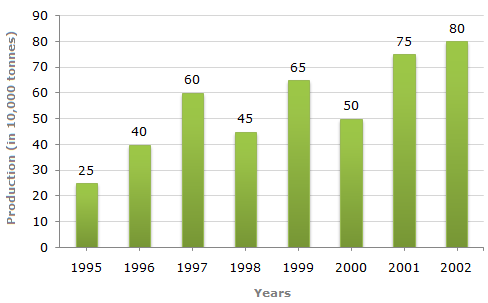# Data Interpretation - Bar Charts - Discussion

Study the bar chart and answer the question based on it.

Production of Fertilizers by a Company (in 1000 tonnes) Over the Years1.

What was the percentage decline in the production of fertilizers from 1997 to 1998?

 [A]. 33(1/3)% [B]. 20% [C]. 25% [D]. 21%

Explanation:

 Required percentage =(45 - 60)% = -25%. 60There is a decline of 25% in production from 1997 to 1998.

 Romita said: (Aug 13, 2011) Why devided by 60 ?

 Don Rd said: (Mar 31, 2014) Because we have to see from the year from which the production is declined means year from which the device started. Here in 1997 production was 60,000 and it started dropping down to 45,000. So whenever declined is to be calculated it has to be calculated with respect to the high value from where the degradation started.

 Raza said: (Aug 18, 2014) Why (45-60)? Why not (60-45)? And can you elaborate the answer for me.

 Shweta said: (Jan 25, 2015) Because of percentage decline ask in question.

 Karthik Kuppa said: (Sep 16, 2016) @Raza. Here there is nothing to elaborate. The question was asked in a negative way. So we have to get the answer in negative value. So we have to do it as 45-60 instead of 60-45. Thank you. :).

 Lakshmi Narayana.P said: (Sep 28, 2016) Why doesn't (60 - 45) take?

 Dhivya said: (May 14, 2018) Why doesn't take (60-45)? Anyone elaborate, please.

 Bhavani said: (May 27, 2019) (60-45) /45 = 1500/45. = 33.3%. The answer is not a negative percentage so because we can calculate interchange the year value because ask in question the decline percentage. The decline means a negative percentage.

 Dileep said: (Jun 20, 2019) Why we take (45-60)/45?

 Sangay Choden said: (Aug 18, 2021) Can anyone elaborate in detail? please.

 Sabyasachi said: (Aug 22, 2021) When they asked for 1997 to 1998 we should take 1998 as a denominator so the answer will be 33.33%.